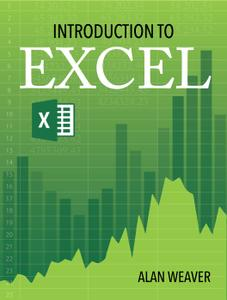# Introduction to Excel

An interesting an engaging introduction to excel for a diversity of learning styles.

# Excel

Excel is a spreadsheet with two basic concepts:

1. A place to crunch your numbers. Think of Excel as a glorified calculator where you can keep changing the numbers – for example if I earn \$10 and hour and work 40 hours a week, what will my salary be if I get 12% raise?

2. Database or lists – you can do a wedding list, club membership, customer list/preferences – create labels, letters, ask questions of the data – narrowing down 22,000 rows down to 4 discernible pieces of data that show what you’re looking for.

This sample lesson - using the file named Daily Planet Payroll has two parts to it.  The first part shows you the basics in creating a formula, working with absolute references, and copying the formula.  The second part demonstrates if statements which allow you to display the answer based on the condition being true or false.  There is a lesson plan included here named Excel sample lesson which you can download.  It includes instructions for this tutorial.  Follow this video to complete the formulas.

You can register for the class with 12 lessons via the following method

insert instructions

Students will receive some sample questions such as the ones below - they will help to test how much you remember from the lesson

Question 1

What did we press or type on the keyboard to begin a basic arithmetic formula (addition, subtraction, division, multiplication)?

A

multiply function [*]

B

equal sign [=]

C

calculate

D

cell reference such as B2

Question 2

When creating an IF statement, there are several conditions to be met...which one does NOT exist?

A

Maybe

B

True

C

False

Question 3

The shortcut key to make an absolute function (i.e. convert D2 to \$D\$2 is

A

press the \$ sign after the cell entry

B

F10

C

F4

D

CTRL + A